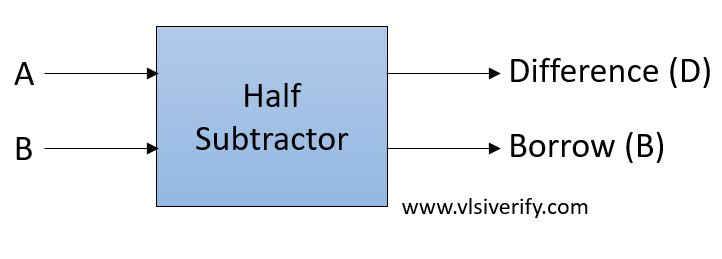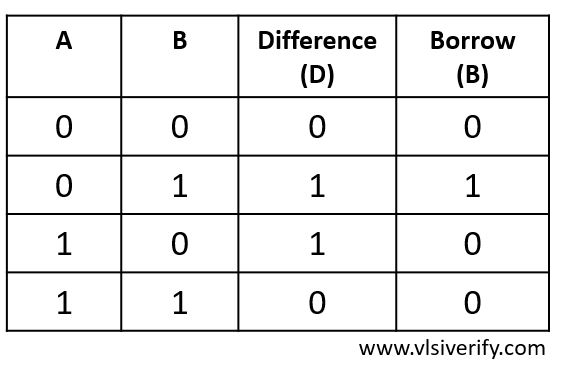Tutorials

The half subtractor works opposite to the half adder as it substracts two single bits and results in a difference bit and borrow bit as an output.

Block DiagramTruth TableOutput:
D = A ^ B
B = A’ · B

As half subtractor considers only two bits so along with the subtraction of two single bits, it can not accommodate an extra borrow bit from the previously generated result. Hence, it is called a half-subtractor. A full subtractor is designed to accommodate the extra borrow bit from the previous stage.

## Half Subtractor Verilog Code

``````module half_subtractor(input a, b, output D, B);
assign D = a ^ b;
assign B = ~a & b;
endmodule``````

### Testbench Code

``````module tb_top;
reg a, b;
wire D, B;

half_subtractor hs(a, b, D, B);

initial begin
\$monitor("At time %0t: a=%b b=%b, difference=%b, borrow=%b",\$time, a,b,D,B);
a = 0; b = 0;
#1;
a = 0; b = 1;
#1;
a = 1; b = 0;
#1;
a = 1; b = 1;
end
endmodule``````

Output:

``````At time 0: a=0 b=0, difference=0, borrow=0
At time 1: a=0 b=1, difference=1, borrow=1
At time 2: a=1 b=0, difference=1, borrow=0
At time 3: a=1 b=1, difference=0, borrow=0``````

Verilog Codes